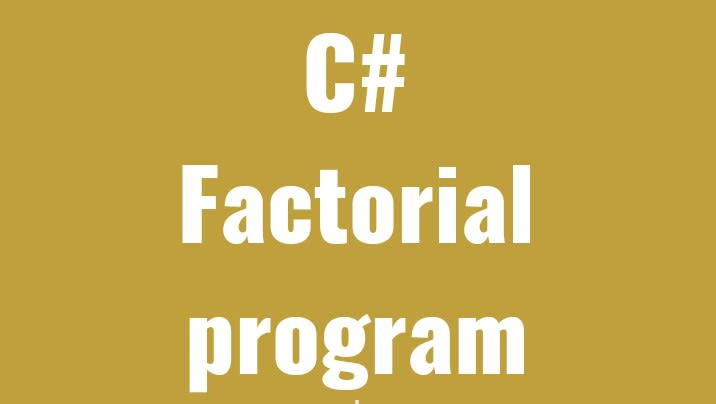# Factorial program c#

Top 10
17 Jul 2020
Give a thumbs up
1

## What is factorial

Factorial is the product of all positive integers less than or equal to a given positive integers (as 4) and denoted by that integer and an exclamation point.

If you want to get 4 factorial, then

Example: factorial of 4

1. 4! = 4*3*2*1 = 24
2. 6! = 6*5*4*3*2*1 = 720

Factorial of 4 = 24

Factorial of 6 = 720

## Write factorial program in c#

1. using Sytem;
2. public class Factorialprogram
3. {
4. public static void main(string[] args)
5. {
6. int I, fact=1,number;
7. Console.Write("Enter any number");
9. for(i=1;i&lt;=number;i++){
10. fact = fact * i;
11. }
12. Consoe.Write("Factorial of " +number+" is: "+fact);
13. }
14. }

## Output:

Enter any number 4

Factorial of 4 is 24Author
##### Top 10
Blogger Top 10 content related post